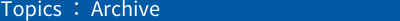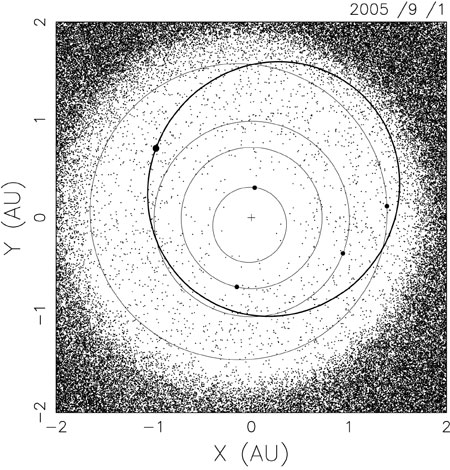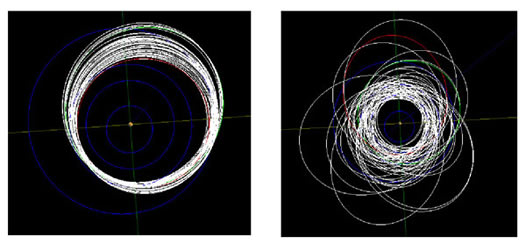TOP > Topics > 2005 > Orbital Evolution of Asteroid Itokawa# Orbital Evolution of Asteroid Itokawa

What orbit did the asteroid Itokawa follow in the past? Where will it go? We have studied the orbital evolution of Itokawa. This study will give some useful information, when we explain the observation results by HAYABUSA.

## 1. Orbit of Itokawa

The present orbit of Itokawa is shown in Fig.1. Its orbit looks like to cross the orbits of the Earth and Mars. Actually the orbital inclination of Itokawa is small so its orbital plane is quite near to that of the Earth (=Ecliptic plane). Therefore, Itokawa approaches the Earth and Mars frequently. In Fig.1, the positions of asteroids whose orbit are determined are plotted. The asteroids plotted inside of the Mars orbit are members of Near Earth Objects (NEO) and they may approach the Earth closely. Itokawa is one of them.

If an asteroid is in an orbit that approach planets, its orbital evolution becomes chaotic. This means that the negligibly small difference in the initial condition will become very large quite soon. There is error in the determined orbit, and by this error we cannot predict its future orbit if the orbital evolution is chaotic. Asteroid Itokawa is indeed in such situation.Fig.1 Orbit of Itokawa. The thick line is the orbit of Itokawa, and other lines are those of Mercury, Venus, Earth, Mars, from inside to outside. The positions of asteroids on 1 September 2005 are also plotted.

## 2. Orbital evolution of Itokawa

When we study orbital evolution of celestial bodies in chaotic state, it is not enough to calculate their orbital motion simply. For example, in the case of Itokawa, the small error in the initial condition grows very rapidly, and after the calculation of about 200 years, the error becomes as large as the scale of the orbit itself. In order to know the orbital evolution for the chaotic motion, we consider many hypothesis objects ("clone"), whose initial orbits are slightly different from the nominal orbit. Then those orbits are calculated and studied by statistical methods.

At first, we studied the orbital evolution of Itokawa in the past by statistical methods. As the results, it is found that Itokawa possibly existed near the present orbit at 5000 years ago.

We can estimate origin of Itokawa by using an steady state model of orbital evolution of near earth objects. The detail things are omitted here, but it is found that Itokawa probably came to the present orbit from a region where a certain kind of resonance occurs or the region where the orbit crosses Mars orbit. Thus we can say the origin of Itokawa may be near the inner part in the asteroid belt. However, it is difficult to know when the orbit of Itokawa became current one. The typical time scale of such orbital change is about several million years. Maybe we can get some clues from the observation by Hayabusa.

Next, we studied the orbital evolution of Itokawa about 100 million years in the future, and we found that the fate of Itokawa ends most probably by a collision with the sun or planets (Mercury, Venus, Earth, Mars). Only in a small probability, it collides to Jupiter, or it is thrown away farther than Saturn, or it is still alive orbiting in the near earth region. The probability of colliding with the earth is about once in one million years. The collision frequency of about Itokawa-size objects (about 500m) is said to be about once in several hundred thousand years, so the collision probability of Itokawa is rather high as only one object.

Fig.2 shows the orbital changes of two cases ("clones"), which finally collide to the earth. Especially for the case of long periods, the orbit is changing very largely. This shows chaotic motion straightforwardly.
(You do not have to worry about the earth collision of Itokawa in near future.)Fig.2 The orbital changes of two "clones" that collide to the earth. These figures show the orbital changes in arbitral time span for the case of the earth collision in about 60,000 years (left) and about 13 million years (right). The orbits in blue lines are those of Mercury, Venus, Earth, and Mars, from inner to outer.

## 3. Summary

The orbital evolution of Itokawa is as follows:

Itokawa existed in the inner part in the asteroid belt, and it was in a certain resonant state or in the region where the orbit crosses that of Mars.

Itokawa is in an orbit that approaches the earth and Mars. The orbit motion is strongly chaotic and we can trace its orbit only for about 200 years.

Itokawa most probably collides to Sun or planets (Mercury, Venus, Earth, Mars). In a small probability, it remains alive more than 100 million years, or its orbit becomes larger than that of Jupiter. The probability of the collision to the earth is about once in one million years.

As the summary, Itokawa has evolved to the current orbit from the inner part of the asteroid belt, and it will collide to the sun or the planets (Mercury, Venus, Earth, Mars). This is the typical evolution of near earth objects. This means that the information that HAYABUSA gets from Itokawa is important for spaceguard as well as the studies of asteroid origin and asteroid-meteorite relationship.

October 26, 2005